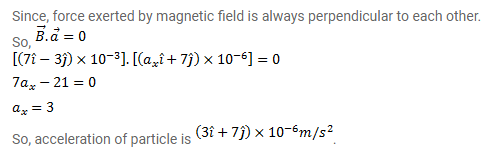# An experiment's diary reads as follows:" a charged particle is projected in a magnetic field of

Question:

An experiment's diary reads as follows:" a charged particle is projected in a magnetic field of $(7.0 \vec{\imath}-3.0 \vec{\jmath}) \times 10^{-3} \mathrm{~T}$. The acceleration of the particle is found to be $(\vec{\imath}+7.0 \vec{\jmath}) \times 10^{-6} \mathrm{~m} / \mathrm{s}^{2 n}$. The number to the left $\bar{i}_{\text {in }}$ the last expression was not readable. What can this number be?

Solution: